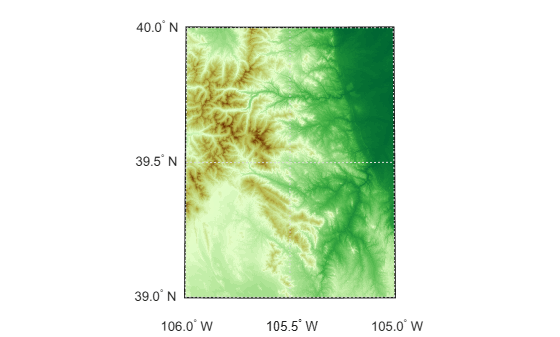# geographicGrid

Geographic coordinates of raster elements

Since R2021a

## Syntax

``[lat,lon] = geographicGrid(R)``
``[lat,lon] = geographicGrid(R,gridOption)``

## Description

example

````[lat,lon] = geographicGrid(R)` returns the geographic coordinates of raster elements as the 2-D arrays `lat` and `lon`. The coordinates of raster element `(i,j)` are `(lat(i,j),lon(i,j))`.```

example

````[lat,lon] = geographicGrid(R,gridOption)`, where `gridOption` is `'gridvectors'`, returns `lat` and `lon` as vectors. The coordinates of raster element `(i,j)` are `(lat(i),lon(j))`. The default for `gridOption` is `'fullgrid'`, which returns `lat` and `lon` as 2-D arrays.```

## Examples

collapse all

Import elevation data  for an area around South Boulder Peak in Colorado as an array and a geographic postings reference object. Find the coordinates of each element in the array.

```[Z,R] = readgeoraster('n39_w106_3arc_v2.dt1'); [lat,lon] = geographicGrid(R);```

Create a map with latitude and longitude limits that match the limits of the data. Display the data using an appropriate colormap.

```usamap(R.LatitudeLimits,R.LongitudeLimits) surfm(lat,lon,Z) demcmap(Z)```The elevation data used in this example is courtesy of the U.S. Geological Survey.

Create a geographic cells reference object for a 3-by-4 raster with latitude values in the range [0, 30] degrees and longitude values in the range [-20, 20] degrees. Get the coordinates of the raster elements and return them as row vectors.

```R = georefcells([0 30],[-20 20],[3 4]); [lat,lon] = geographicGrid(R,'gridvectors')```
```lat = 1×3 5 15 25 ```
```lon = 1×4 -15 -5 5 15 ```

If you do not specify the second argument as `'gridvectors'`, then the `geographicGrid` function returns 2-D arrays by default.

`[latFull,lonFull] = geographicGrid(R)`
```latFull = 3×4 5 5 5 5 15 15 15 15 25 25 25 25 ```
```lonFull = 3×4 -15 -5 5 15 -15 -5 5 15 -15 -5 5 15 ```

## Input Arguments

collapse all

Spatial reference, specified as a `GeographicCellsReference` or `GeographicPostingsReference` object.

If `R` is a `GeographicCellsReference` object, then `lat` and `lon` are cell centers. If `R` is a `GeographicPostingsReference` object, then `lat` and `lon` are posting points.

Grid option, specified as one of these values:

• `'fullgrid'` — Return `lat` and `lon` as 2-D arrays, where each column of `lat` is identical and each row of `lon` is identical. This is the default behavior.

• `'gridvectors'` — Return `lat` and `lon` as row vectors. Use this option when you want to reduce memory usage and when 2-D arrays are unnecessary, such as when plotting large data sets with the `surfm` function.

This table shows the difference between `'fullgrid'` and `'gridvectors'`.

`'fullgrid'``'gridvectors'`
```R = georefcells([0 30],[-20 20],[3 4]); [lat,lon] = geographicGrid(R)```
```lat = 5 5 5 5 15 15 15 15 25 25 25 25 lon = -15 -5 5 15 -15 -5 5 15 -15 -5 5 15```
```R = georefcells([0 30],[-20 20],[3 4]); [lat,lon] = geographicGrid(R,'gridvectors')```
```lat = 5 15 25 lon = -15 -5 5 15```

Data Types: `char` | `string`

## Output Arguments

collapse all

Latitudes, returned as a 2-D array or a row vector. By default, `lat` is a 2-D array. To return `lat` as a row vector, specify `gridOption` as `'gridvectors'`.

By default, and when `gridOption` is `'fullgrid'`, the sizes of `lat` and `lon` each equal the `RasterSize` property of `R`. When `gridOption` is `'gridvectors'`, the lengths of `lat` and `lon` equal the first and second elements of the `RasterSize` property of `R`, respectively.

Longitudes, returned as a 2-D array or a row vector. By default, `lon` is a 2-D array. To return `lon` as a row vector, specify `gridOption` as `'gridvectors'`.

By default, and when `gridOption` is `'fullgrid'`, the sizes of `lat` and `lon` each equal the `RasterSize` property of `R`. When `gridOption` is `'gridvectors'`, the lengths of `lat` and `lon` equal the first and second elements of the `RasterSize` property of `R`, respectively.

## Version History

Introduced in R2021a